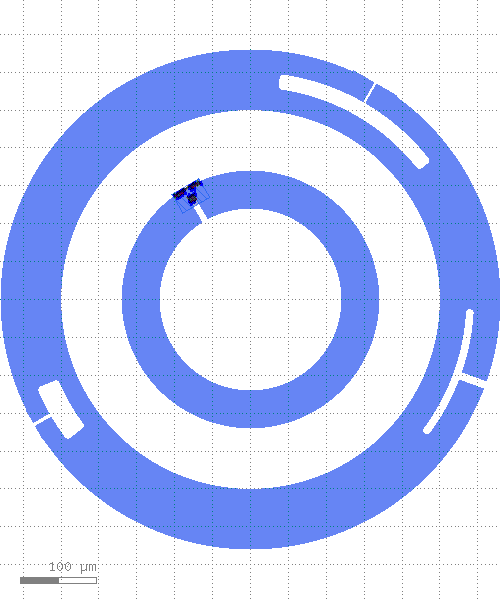# kqcircuits.qubits.concentric_transmon¶

class kqcircuits.qubits.concentric_transmon.ConcentricTransmon[source]

The PCell declaration for a concentric transmon. A concentric transmon consists of two islands, one inner and one outer, connected by a Josephson Junction/s. Multiple couplers can be defined. They can have custom waveguide impedance, size and shape. Each coupler has reference points, numbered starting from 1. Driveline can be connected to the drive port.

build()[source]

Child classes re-define this method to build the PCell.

classmethod get_sim_ports(simulation)[source]

List of RefpointToSimPort objects defining which refpoints should be turned to simulation ports for the given element class

Returns empty list if not implemented for Element subclass. When implementing this method, the best practice is for this method to have no “side effects”, that is all code contained within this method should only serve to derive the list of RefpointToSimPort objects and nothing else: no change in element’s geometry or parameter values.

Parameters
• cls – Element class, this is a class method

• simulation – Simulation object where a cell of this element class is placed. Use this argument if you need to decide certain arguments for RefpointToSimPort objects based on simulation’s parameters

Returns

List of RefpointToSimPort objects, empty list by default

PCell parameters:

• r_inner (Double) - Radius of the outer edge of the inner island, default=`120`, unit=`μm`

• r_outer (Double) - Radius of the external qubit island, default=`250`, unit=`μm`

• outer_island_width (Double) - Width of the external island, default=`80`, unit=`μm`

• ground_gap (Double) - Ground plane padding, default=`80`, unit=`μm`

• squid_angle (Double) - Angular position of the Josephson Junction/s, where the positive x-axis, default=`120`, unit=`degrees`

• couplers_r (Double) - Radius of the couplers positioning, default=`290`, unit=`[μm]`

• couplers_a (List) - Width of the coupler waveguide’s center conductors, default=`[10, 3, 4.5]`, unit=`[μm]`

• couplers_b (List) - Width of the coupler waveguide’s gaps, default=`[6, 32, 20]`, unit=`[μm]`

• couplers_angle (List) - Positioning angles of the couplers, where 0deg corresponds to positive x-axis, default=`[340, 60, 210]`, unit=`[degrees]`

• couplers_width (List) - Radial widths of the arc couplers, default=`[10, 20, 30]`, unit=`[μm]`

• couplers_arc_amplitude (List) - Couplers angular extension, default=`[35, 45, 15]`, unit=`[degrees]`

• drive_angle (Double) - Angle of the drive port, where 0deg corresponds to positive x-axis, default=`300`, unit=`degrees`

• drive_distance (Double) - Distance of the driveline, measured from qubit centre, default=`400`, unit=`µm`

• n (Int) - Number of points on turns, default=`180`